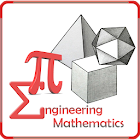Engineering mathematics

All Android applications categories

All Android games categories# Engineering mathematics

808 8.2

8.2 Users
rating

## Screenshots

Description

The app is a complete free handbook of Engineering mathematics with diagrams and graphs. It is part of engineering education which brings important topics, notes, news & blog on the subject. Download the App as quick reference guide & ebook on this Engineering mathematics subject.

It covers 80 topics of Maths in detail. These 80 topics are divided in 5 chapters.

It provides quick revision and reference to the topics like a detailed flash card. Each topic is complete with diagrams, equations and other forms of graphical representations for easy understanding.

The application serves to both engineering students and professionals. Some of topics Covered in this application are:

1. Leibnitz Theorem
2. Problems on Leibnitz Theorem
3. Differential Calculus-I
4. Radius of Curvature
5. Radius of Curvature in Parametric Form
6. Problems on Radius of Curvature
7. Radius of Curvature in Polar Form
8. Cauchy’s Mean Value Theorem
9. Taylor’s Theorem
10. Problems on Fundamental Theorem
11. Partial Derivatives
12. Euler Lagrange Equation
13. Curve Tracing
14. Change of Variable Theorem
15. Problems on Differential Calculus I
16. Indeterminate Forms
17. Problems on L' Hospital Rule
18. Various Indeterminate Forms
19. Problems on Various Indeterminate Forms
20. Taylor’s Theorem For Function of Two Variables
21. Problems on Taylor's Theorem
22. Maxima And Minima of Function of Two Variables
23. Problems Maxima And Minima of Function of Two Variables
24. Lagrange’s Method of Undetermined Multipliers
25. Problems on Lagrange’s Method of Undetermined Multipliers
26. Polar Curves
27. Problems on Polar Curve
28. Jacobian of Transformation
29. Extrema of Function of Several Variables
30. Problems on Differential Calculus II
31. Multiple Integrals
32. Problems on Multiple Integral
33. Double Integral by Changing the Order of Integration
34. Applications to Area and Volume
35. Problems on Applications to Area and Volume
36. Beta And Gamma Function
37. Relationship between Beta and Gamma Functions
38. Problems on Beta and Gamma Functions
39. Dirichlet Integral
40. Dirichlet Integral and Fourier Series
41. Problems on Dirichlet Integrals
42. Triple Integrals
43. Triple Integrals using Cylindrical Coordinates
44. Problems on Integrals
45. Objective Question on Integrals
46. Vector Functions
47. Vector Line Integral
48. Green's Theorem
49. Gauss Divergence Theorem
50. Stoke Theorem
51. Surface and Volume Integrals
52. Problems on Integrals Theorem
53. Directional Derivative of Vector
54. Vector Gradient
55. Theorem of Line Integral
56. Orthogonal Curvilinear Coordinates
57. Differential Operators
58. Divergence of Vector
59. Curl of Vector
60. Problems on Vector Calculus
61. Introduction of Matrices
62. Properties of Matrices
63. Scalar Multiplication
64. Matrix Multiplication
65. Transpose of Matrix
66. Nonsingular Matrix
67. Echelon Form of Matrix
68. Determinant
69. Properties of Determinants
70. System of Linear Equation
71. Solution to a Linear System
72. Solution to Linear System by Inverse Method
73. Rank and Trace of Matrix
74. Cayley-Hamilton Theorem
75. Eigenvalues and Eigenvector
76. Method of Finding Eigenvalues and Eigenvectors
77. Diagonalisation of Matrices
78. Unitary Matrices
79. Idempotent Matrices
80. Problems on Matrices

from 808 reviews

"Great"

8.2